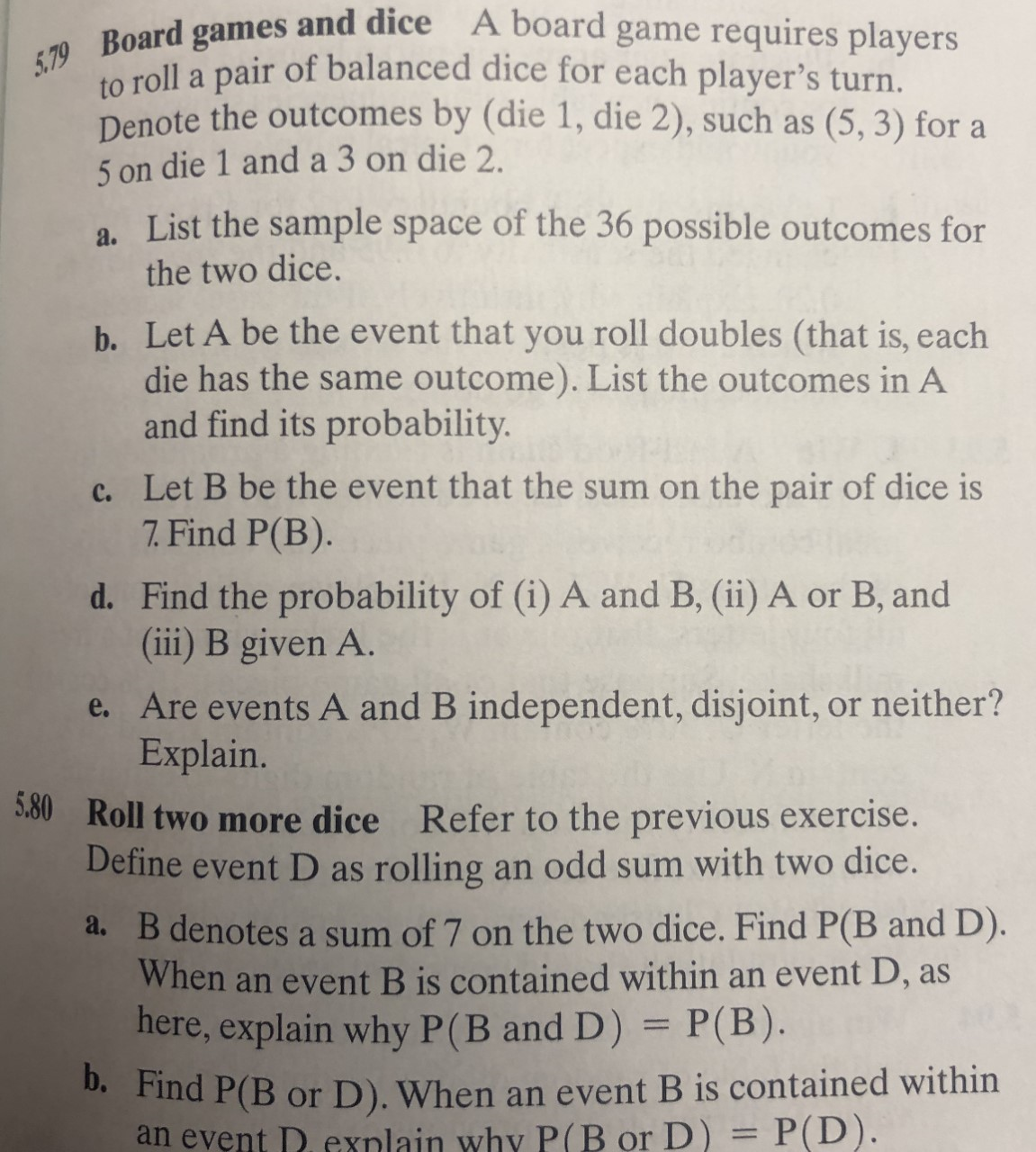# 5.79 Board games and dice A board game requires playersto roll a pair of balanced dice for each player's turn.Denote the outcomes by (die 1, die 2), such as(5, 3) for a5 on die 1 and a 3 on die 2.a. List the sample space of the 36 possible outcomes forthe two dice.b. Let A be the event that you roll doubles (that is, eachdie has the same outcome). List the outcomes in Aand find its probability.c. Let B be the event that the sum on the pair of dice is7.Find P(B).d. Find the probability of (i) A and B, (ii) A or B, and(iii) B given A.e. Are events A and B independent, disjoint, or neither?Explain.530Roll two more dice Refer to the previous exercise.Define event D as rolling an odd sum with two dice.a. B denotes a sum of 7 on the two dice. Find P(B and D).When an event B is contained within an event D, ashere, explain why P(B and D) = P(B).b. Find P(B or D). When an event B is contained withinan event D.exnlain why P(B or D) = P(D).

Question
1 views

5.80 but use 5.79 as a referencehelp_outlineImage Transcriptionclose5.79 Board games and dice A board game requires players to roll a pair of balanced dice for each player's turn. Denote the outcomes by (die 1, die 2), such as (5, 3) for a 5 on die 1 and a 3 on die 2. a. List the sample space of the 36 possible outcomes for the two dice. b. Let A be the event that you roll doubles (that is, each die has the same outcome). List the outcomes in A and find its probability. c. Let B be the event that the sum on the pair of dice is 7.Find P(B). d. Find the probability of (i) A and B, (ii) A or B, and (iii) B given A. e. Are events A and B independent, disjoint, or neither? Explain. 530Roll two more dice Refer to the previous exercise. Define event D as rolling an odd sum with two dice. a. B denotes a sum of 7 on the two dice. Find P(B and D). When an event B is contained within an event D, as here, explain why P(B and D) = P(B). b. Find P(B or D). When an event B is contained within an event D.exnlain why P(B or D) = P(D). fullscreen
check_circle

Step 1

Consider,

D be the event that shows an odd sum on rolling of two dice.

B be the event that denotes a sum of 7 on the two dice.

Step 2

A)

The required probability is-

D = {(odd, even) or (even, odd)},

B = {(3, 4), (4,3), (5,2) ,(2,5),(6,1),(1,6)}.

Step 3

Since, B is the subset of D that is D contains the B.

T...

### Want to see the full answer?

See Solution

#### Want to see this answer and more?

Solutions are written by subject experts who are available 24/7. Questions are typically answered within 1 hour.*

See Solution
*Response times may vary by subject and question.
Tagged in

### Probability